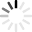# Coordinate Geometry Quizzes & Trivia

Coordinate geometry is the study of geometry using a coordinate system. Are you good at lining things up? If so, then line up theses fun quizzes about coordinate geometry. Pinpoint questions like: “In classical mathematics, what is coordinate geometry also referred to as?”, “Is coordinate geometry used in physics?”, “How does coordinate geometry involve linear algebra?”, “What is a vector space?”, and “What is synthetic geometry and how does it contrast with coordinate geometry?”.

In the end, the fun will line up easily with these quizzes. Whether you have expert knowledge on coordinate geometry or not, you will enjoy yourself with these quizzes. As long as you remember to have fun things will line up easily. Good luck with this!
Top Trending

Questions: 15  |  Attempts: 2043   |  Last updated: Dec 21, 2020
• Sample Question
The distance of the point P (2,3) from the x-axis is:Coordinate geometry is a branch of mathematics representing the points, lines, and geometric figures in the coordinate system and studying their geometric properties. It helps with solving many problems that come up in common...

Questions: 10  |  Attempts: 440   |  Last updated: Apr 21, 2021
• Sample Question
If (4,1) is the midpoint of the interval from (x,-2) to (5,y), what is the value of y?Could you pass this quiz on coordinate geometry? Coordinate geometry describes the connection between geometry and algebra using graphs that are comprised of curves and lines. It gives you the perspective of geometric...

Questions: 15  |  Attempts: 927   |  Last updated: Apr 21, 2021
• Sample Question
The points (–4,–8) lies in:Coordinate Geometry ACT Questions

Questions: 15  |  Attempts: 409   |  Last updated: May 30, 2016
• Sample Question
In the standard (x,y) coordinate plane below, the points (0,2), (8,2), (3,6) and (11,6) are teh vertices of a parallelogram. What ist he area, in square units, of the parallelogram?Difficult coordinate geometry ACT questions

Questions: 9  |  Attempts: 937   |  Last updated: Jan 16, 2013
• Sample Question
Given the vertices of parallelogram QRST in the standard (x, y) coordinate plane below, what is the area of triangle QRS, in square units?Related